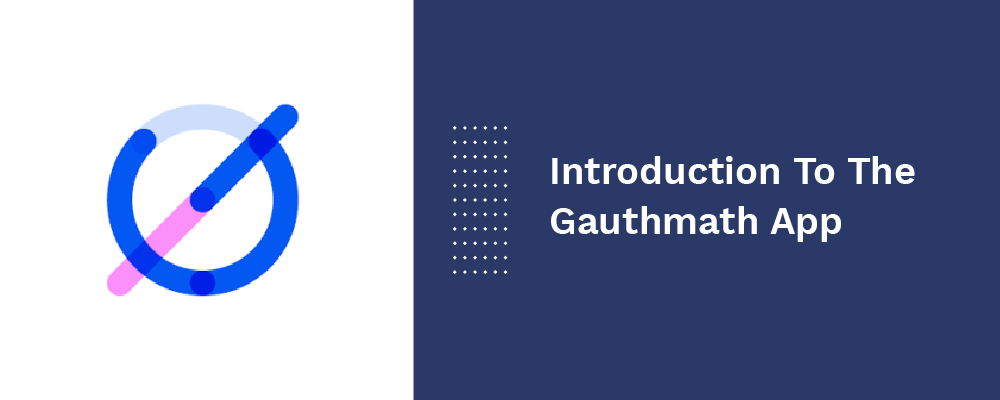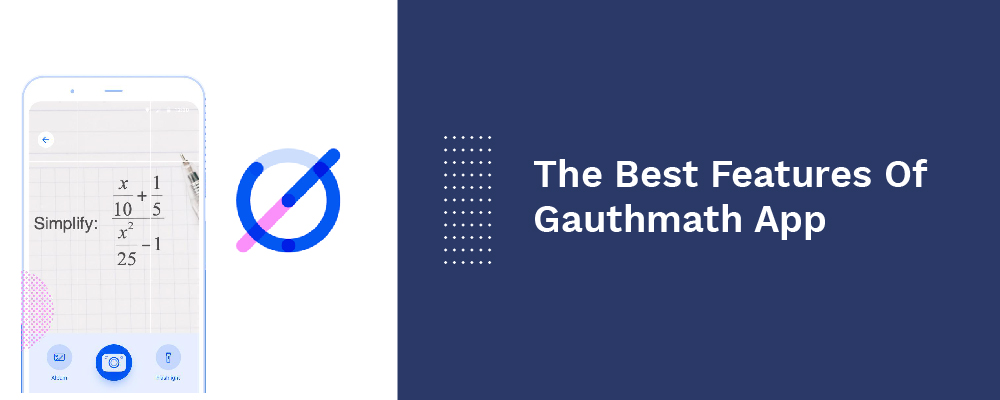+44 20 8133 8639

As much as challenging maths looks from the outside, it isn't. It all depends on the teaching style. All cannot be good at maths; it depends on the level of interest to learn. For working parents, it's not possible to be available for their kids all the time to help them weather math anxiety. So, how can you help your children struggling with intricate math problems?

While teaching maths, you must have tried everything from quizzes to additional study help. However, you can easily bring math professionals live to your home through smartphones, through a fantastic app called Gauthmath. It's a free online math problem solver to help you learn maths online, including topics such as track formula, algebra, geometry, and solving equations by pointing the camera.

In this blog, we will look at this unique maths problem solve app and how handy it can be for someone anxious about math.

Introduction To The Gauthmath AppGauthmath app is the ultimate free online math problem solver that reads & solves math problems promptly using the camera of your mobile phone. From basic computations to advanced calculus, this app uses smart tech to read math issues and render stepwise solutions to address them.

This math problem solver app is reasonably the top app for solving arithmetic problems that provide free portable tutors for calculus, graphing, algebra, and other complex math problems, anywhere, anytime. You only need to take a picture of your question and get stepwise answers instantly within 3 mins of posting.

As per the Gauthmath team, "Gauthmath solves word problems which almost no math solver app could do. Because we have real live tutors, with an efficient question sending order system, students from worldwide could get their questions answered within a short time by a well-trained tutor."

When Do You Need This Online Math Problem Solve App?

In case you have any end-minute math problems, leave them to Gauthmath! This app really is great and usable to solve math arithmetic equations. It is super handy when kids have no one around for a tricky homework question. For example, students have a math question but no one to help them with it, all they gotta do is snap the question & a tutor from Gauthmath would offer them a stepwise answer within 1 to 15 min for free. It works excellent for math athletes or math-challenged kids dealing with end-minute problems.

This math helper app spares a lot of time for students from watching pre-recorded videos, rather than rendering them with fast explanations to problems to the point. Secondly, the answers given by these tutors are most precise with details. There are also certified experts ready to assist you 24*7, which is a considerable benefit that AI could rarely solve due to NLP tech.

The Best Features Of Gauthmath AppThis free online math helper app has various features making it the top math solver app to learn maths online.

1. Solve All Arithmetic Problems In One App

Gauthmath can help you solve your math homework with your mobile phone. You just need to take a photo of the math equation or scan your maths homework, and the app does the rest of things. This math solver app will directly get you the solution, whether it is calculus, algebra, graphing, or other intricate math equations.

2. Get Real-time Homework Assistance

The problem solver app reads & solves arithmetic problems promptly by using the mobile's camera. Once you take a picture of your question, it barely takes 3 mins or less to find the solution. The answer to the problem exhibits on the screen instantly.

3. Get Step-by-Step Explanations

From fundamental to advanced calculus and geometry, this app gives in-depth explanations in the simplest form. Unlike other math solver apps, this one doesn't jump to response but promptly provides a stepwise solution to your math problem.

4. Certified Experts Ready To Assist You 24*7

This app has tons of math professionals & free portable tutors. Once they receive your math problem picture, you get answers promptly, and stepwise solutions will be offered to you if required. Just ask them, anytime, anywhere, and even 24*7.

5. The World's Simplest Math Scanner

This app can help you solve the most challenging types of math problems, such as IB/SAT/ACT/A Level/AP/GCSE/HSC math and other advanced maths. It covers all sorts of arithmetic problems that help you master them.

Math Topics Included

It offers content from every significant problem and includes the topics below:

• Word problems
• Function (Quadratic; Polynomial; Linear; Exponential; Logarithmic; Inverse Function; Rational)
• Algebra (Real Number; Expression; Arithmetic; Logarithm; Complex Number; Set theory)
• Geometry (Plane & Solid Geometry; lines & planes in space; Algebra & lines; transformation)
• Calculus (Integrals; Derivatives; the tangent/area problem; Limits)
• Trigonometry (Law of Sines; Trigonometric Ratios; Law of Cosines; Reciprocal Properties)
• Matrix (The system of linear equations & matrices; matrix algebra)
• Statistics & Data Analysis (Data representation; random variables; Probability; binomial theorem; normal distributions; confidence intervals; Poisson distribution)
• Logic (Conditional statements; inductive reasoning; reasoning & proof; deductive reasoning)

Pros & Cons

Pros

• Solve math problems quickly
• 100% free to use
• Live math professionals available 24*7
• Free & lightweight app
• Stepwise explanations
• Endless chances to ask math professionals
• User-friendly interface

Cons

• The interface can be enhanced
• Video explanations are missing
• It doesn't support handwriting

The Endnote

New tech judges how a student learns, their habits, and how their goals can be attained. This concept gives rise to the Gauthmath app. It's a free-to-use app for Android and iOS users that aim to revolutionize education & learning. This app is reasonably the top app for solving math problems with professional help. It's a really unique tool that will become very successful amongst parents, students, and even teachers to resolve math problems while staying at home.

We also discovered that the app is phenomenal and handy to solve maths equations without hassle. Download the Gauthmath app on your mobile phone and start learning smartly. If you are a business owner and want to create a similar app, get in touch with us to guide you further.